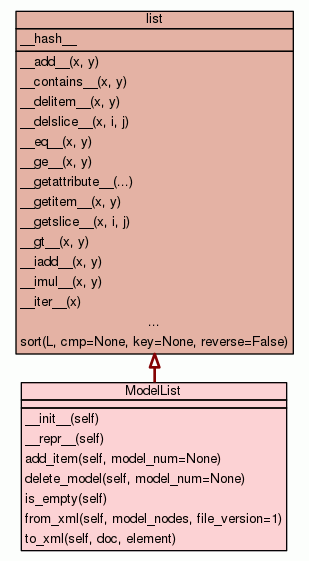[frames] | no frames]

# Class ModelList

source codeList type data container for the different structural models.

Here different models are defined as the same molecule but with different conformations.

 Instance Methods
new empty list
 __init__(self) Set up the class. source code

 __repr__(self) The string representation of the object. source code
ModelContainer instance
 add_item(self, model_num=None) Append an empty ModelContainer to the ModelList. source code

 delete_model(self, model_num=None) Delete the given model from the list. source code
bool
 is_empty(self) Method for testing if this ModelList object is empty. source code

 from_xml(self, model_nodes, file_version=1) Recreate a model list data structure from the XML model nodes. source code

 to_xml(self, doc, element) Create XML elements for each model. source code

Inherited from `list`: `__add__`, `__contains__`, `__delitem__`, `__delslice__`, `__eq__`, `__ge__`, `__getattribute__`, `__getitem__`, `__getslice__`, `__gt__`, `__iadd__`, `__imul__`, `__iter__`, `__le__`, `__len__`, `__lt__`, `__mul__`, `__ne__`, `__new__`, `__reversed__`, `__rmul__`, `__setitem__`, `__setslice__`, `__sizeof__`, `append`, `count`, `extend`, `index`, `insert`, `pop`, `remove`, `reverse`, `sort`

Inherited from `object`: `__delattr__`, `__format__`, `__reduce__`, `__reduce_ex__`, `__setattr__`, `__str__`, `__subclasshook__`

 Class Variables

Inherited from `list`: `__hash__`

 Properties

Inherited from `object`: `__class__`

 Method Details

### __init__(self)(Constructor)

source code

Set up the class.

Returns: new empty list
Overrides: object.__init__

### __repr__(self)(Representation operator)

source code

The string representation of the object.

Rather than using the standard Python conventions (either the string representation of the value or the "<...desc...>" notation), a rich-formatted description of the object is given.

Overrides: object.__repr__

source code

Append an empty ModelContainer to the ModelList.

Parameters:
• `model_num` (int) - The model number.
Returns: ModelContainer instance
The model container.

### delete_model(self, model_num=None)

source code

Delete the given model from the list.

Parameters:
• `model_num` (int) - The model to delete.

### is_empty(self)

source code

Method for testing if this ModelList object is empty.

Returns: bool
True if this list only has one ModelContainer and the model name has not been set, False otherwise.

### from_xml(self, model_nodes, file_version=1)

source code

Recreate a model list data structure from the XML model nodes.

Parameters:
• `model_nodes` (xml.dom.minicompat.NodeList instance) - The model XML nodes.
• `file_version` (int) - The relax XML version of the XML file.

### to_xml(self, doc, element)

source code

Create XML elements for each model.

Parameters:
• `doc` (xml.dom.minidom.Document instance) - The XML document object.
• `element` (XML element object) - The element to add the model XML elements to.

 Generated by Epydoc 3.0.1 on Fri Jun 14 11:29:42 2019 http://epydoc.sourceforge.net